RegisterLoginForumSearchFAQ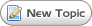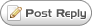Page 1 of 2 [ 13 posts ] Go to page 1, 2  Next

#### Optimising the stoplossPrint view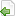Previous topic | Next topic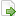#### Optimising the stoploss

Author Message
 Post subject: Optimising the stoplossPosted: Wed Mar 02, 2011 9:10 pmJoined: Fri Nov 05, 2010 8:31 am
Posts: 72
I thought I would stir up the Money management forum again with another one of my long thoughts:
After running the backtests on the joint strategy, which basically showed that changing the stoploss policy made a lot difference, I have been trying to work out a good way to assess whether the stop loss is in the right place.
I have been doing lots of thinking about this, which led me on to the idea that one possible way of assessing the placement of the stop loss is to do some statistical analysis of where tomorrows low price (for longs only at the moment) is in relation to todays prices.
Thus I have set up a test which trawls through the FTSE 350 stocks for last year and it calculates the difference in price between some combination of todays data and the low for tomorrow.
If we were able to predict the position of tomorrows low accurately we would end up with a set of differences which would all be very small. Obviously I’d be a millionaire if I could do that accurately! However I can test how well my prediction is, by looking at the statistical dispersion of the results, i.e. the lower the dispersion the better we are at predicting tomorrow low.
What we want is to end up with is a distribution of “errors” which has a low standard deviation, and a high Kurtosis. ( Kurtosis is a measure of how “peaked” a distribution is compared to a normal distribution). I can then change the methods which I am using to predict where tomorrows low is and thus compare one method against another. What I am hoping is that this will allow us to make a sensible choice as to whether it is best to set the stop loss from typical price, close ,low , low of the last n bars, a price minus K times AVTR , etc etc.
That was the basic idea of what I want to do, in the implementation I wanted to make sure that I took volatility into account since I thought that the errors might be more determined by volatility than errors in the predictions. Thus I divided the difference between the prediction and tomorrows low by the AVTR (10 initially but I might try others) This gives me an error which is a percentage of AVTR and thus should be a good comparison across different stocks and different times of volatility.
So the calculations that I did are :
For each stock in the FTSE 350 for every day in 2010, I calculated the difference between my prediction and tomorrows low, divide this by todays AVTR, then for each set of these values I calculated the Average, Standard Deviation, Skew and Kurtosis, to arrive at a set of 350 values for each one of these, (one for each stock). I then calculated the Average, Standard Deviation, Skew and Kurtosis of each column of figures. ( I know abut the dangers of averaging averages but I was just trying to compare one method with another). I then repeated these test using different methods for calculating the predicted low. The methods I used were:
Reference Prices: Close, Typical Price, Low, Low of the last 3 days
AVTR Offset from each of these prices times K , using different values of K
Using different number of days for the AVTR calculation
The initial results are interesting in that they do show a considerable variation in the Standard deviation of the Standard devations ( if you see what I mean) .( Maximum 0.935, minimum 0.56) The method that is consistently lower is Close – K *AVTR, This method shows good positive Kurtosis of the Standard deviation distribution and also show good positive average Kurtosis. Using Low of the Last N bars is consistently worse than the other methods. I still need to run more tests to find the optimum values, but since each run takes a while , it will take me some time to complete this.
If anybody has any comments or improvements that they feel like suggesting I would welcome it, since I am not a statistician and I could easily have fallen down a hole.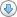Post subject: Re: Optimising the stoplossPosted: Thu Mar 03, 2011 9:09 amJoined: Wed Nov 24, 2010 8:33 pm
Posts: 15
Your statistical mathematics have gone right over my head, but I look forward to seeing the end results.

On a personal note I was trying the turtle rules out on ITV and have fallen foul of the 2 week low stop out at 81p only to see the stock rocket up again into the nineties. Not very happy so I can see where the low of the N days may be a loser.

cheers
Andrew.Post subject: Re: Optimising the stoplossPosted: Thu Mar 03, 2011 10:02 amJoined: Fri Nov 05, 2010 8:31 am
Posts: 72
I have found the first error in my analysis myself, I have decided that I should be using the Median instead of the Average, since if I can predict where the median is I then know that there is a 50-50 chance of it being hit. Using the average won't do that. So I am just rerunning the tests again. What I didn't explain in my first thoughts is that my assumption is that if I can predict the point which is the centre (Not a statistical term!) of the distribution of errors and I know the distribution of errors about that point, if I assume a normal distribution I can predict the probability of any price being hit. If my Kurtosis is greater than a normal distribution then the chances will always be less than that of a normal distribution.
My idea is to calculate this and then try it on the data from Dec 2010 til now to see if I can correctly predict the percentage of stops hit.Post subject: Re: Optimising the stoplossPosted: Thu Mar 03, 2011 9:13 pmJoined: Fri Nov 19, 2010 7:24 pm
Posts: 91
Interesting stuff OTL, how long does it take for your computer to backtest the data? (Dec '10 onwards).
On a side note, is everyone on here a software engineer? Is there something about spread betting that attracts you all_________________Post subject: Re: Optimising the stoplossPosted: Thu Mar 03, 2011 11:47 pmJoined: Fri Nov 05, 2010 8:31 am
Posts: 72
The length of time that each run takes does vary, the minimum was about 6 minutes, but this went up to about 30 minutes for one of the tests when I selected a 50 Day AVTR. When I calculate the AVTR I am using a function that I wrote myself which calculates OK but obviously I could probably do something to speed it up.
As to why SB attracts software engineers, my only thoughts are that software engineers like developing systems and solving problems and of course spread betting is full possibilities for doing that. I really enjoy trying to solve problems like this analysis of stoplosses, and lots of other problems which come up in SB. I have this vague idea that being able to do all this software might give me some advantage, all I can say is hasn't to any great extent so far!! But I have enjoyed the journey.Post subject: Re: Optimising the stoplossPosted: Fri Mar 04, 2011 9:47 amJoined: Fri Nov 05, 2010 8:31 am
Posts: 72
I got some very encouraging results from my tests this morning: having completed a load of tests to investigate what appeared to give the best results over 2010, I then used these figures to predict what percentage of stoplosses would get hit over the first two months of 2011.
The figures below show the following for 2010 set up period and the 2011 test period:
Stop loss not hit tomorrow with stop loss set at my median value:
Stoploss not hit tomorrow with stop loss set 1 standard deviation away from median

The results are very consistent:
2010 Stoploss not hit
50.24% at median
14.68% at 1 std away
2011 Test period Stoploss not hit
53.28% at median
16.60% at 1 STD away
I will give a more detailed analysis of this when I get time to write it. Including more of the figures which have come out of my tests.Post subject: Re: Optimising the stoplossPosted: Fri Mar 04, 2011 12:15 pmJoined: Fri Nov 19, 2010 7:24 pm
Posts: 91
This is good stuff, very interesting numbers.
Will be waiting here to see more analysis! I'd be interested in seeing if its better in the end to work on higher timescales based on a larger percentage stoplosses.

_________________Post subject: Re: Optimising the stoplossPosted: Mon Mar 07, 2011 4:43 pmJoined: Fri Nov 05, 2010 8:31 am
Posts: 72
Hi SBT
I agree with you about it being important to try it for longer terms, but I thought I would try it for the simplest case first, to see whether there is any merit in it, The longer term gets more complex because I woud expect the distibution to get wider and wider and thus small differences in values could create a large difference in probability. I haven't yet worked out exactly how to test the longer term effectively.Post subject: Re: Optimising the stoplossPosted: Mon Mar 07, 2011 6:43 pmJoined: Fri Nov 05, 2010 8:31 am
Posts: 72
For those that are interested in the Results of my testing, I have done full testing on the setting which the initial testing showed to be the best this is where I estimate the median of the next days low from the equation:
Estimated Low = Close -0.39 * AVTR(25)
I then calculate the error between this prediction and tomorrows low and divide this by AVTR (25). I did this for all of 2010 and generated a probability distribution of the errors, I then did the same for 2011 up until now. The results look pretty good. The error in the probability was a maximim 0.75% at any one place, this integrates up to a maximum sum of the errors of 4.5%, however that was above the median where one is not likely to set the stoploss. I was intending to publish the results by uploading the spreadsheet to here but:

I just tried to upload the spread sheet showing all these details but it would appear the xls, files are not allowed, so I will get the results published by some other means.

What these results show is that given today’s close and the AVTR(25) I can calculate the probability that a specific stoploss price will be hit tomorrow with reasonable accuracy.
One might ask why one would want to know this, and I will explain my thinking.
In spread betting, people usually talk about Risk and define it as the number of pounds you are going to lose if your Spread bet stops out.
I personally do not think of risk in this way because it doesn’t take any account of the probability of the stop loss being hit. As one example: if you take a 1£/point long bet on the FTSE100 , without a stoploss you have about £6000 “at risk”, however if you took a £3000/point bet on the FTSE and put the stop loss 2 points away you would also have £6000 at risk. However I don’t need to point out that the chances of your 2 point stoploss being hit is rather higher than the FTSE going to zero, thus the probability of the event occurring does have a big influence on what your losses might be.
The way I calculate the risk :
The probability that an event occurs times the cost if the event occurs.
Thus my desire to work out what the probability of my stop loss getting hit.
As an example:
My probability data shows that the probability of hitting a stop loss set (0.39 + K) AVTR(25) for three values of k is as follows:
K= -0.16 Probability 33%
K=-024 Probabilty 24%
K= -0.4 Probabilty 12.5%

Using my calculation of the Risk in each of these three cases the Risk is:
-0.16 * 33 = 5.4
-0.24 * 24 = 6.3
-0.4 * 12.5 = 5.1
Which shows that setting your stoploss a -0.4 is less risky than setting your stoploss at -0.25

What I am really looking for is the point of the Risk curve that gives the minimum risk as calculated using my risk calculation. Looking at the curves for risk for 2010 and 2011 it remains fairly small and moves up and down a bit as we move the stoploss in from – 4 AVTR. It only gets above 1 % when we get to 1.0 * AVTR. ( remember all of this is offset by 0.39 AVTR from close, so this is effectively setting the stoploss at about 1.4* AVTR (25))
As we move in from here the risk increases rapidly, it has doubled at AVTR 0.8 and is 8 times by the time we get to AVTR 0.5
At less than -0.24 the risk decreases again, this is to be expected because although the probability has got high , the cost of the loss has become small.Post subject: Re: Optimising the stoplossPosted: Mon Mar 07, 2011 8:50 pmJoined: Fri Nov 19, 2010 7:24 pm
Posts: 91
This is good stuff, really appreciate you taking the time to post. I've had about 9 hours sleep in the last 3 days so Im off to bed early and will revisit tomorrow. Just a quick question which will probably be really obvious when I re-read tomorrow, is your ATR(25) taken from EOD data (daily)? 1.4xATR still seems pretty close to me, from your post are you saying 4xATR seems optimal?

_________________Display posts from previous:  Sort byPage 1 of 2 [ 13 posts ] Go to page 1, 2  Next

#### Who is online

 Users browsing this forum: No registered users and 1 guest

 You cannot post new topics in this forumYou cannot reply to topics in this forumYou cannot edit your posts in this forumYou cannot delete your posts in this forumYou cannot post attachments in this forum

Search for: